Next: Hamiltonian Dynamics Up: Lagrangian Dynamics Previous: Spherical Pendulum

Exercises

1. A horizontal rod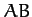rotates with constant angular velocity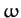about its midpoint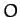. A particleis attached to it by equal strings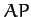,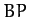. Ifis the inclination of the plane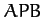to the vertical, prove that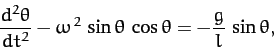where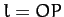. Deduce the condition that the vertical position of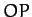should be stable.

2. A double pendulum consists of two simple pendula, with one pendulum suspended from the bob of the other. If the two pendula have equal lengths,, and have bobs of equal mass,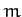, and if both pendula are confined to move in the same vertical plane, find Lagrange's equations of motion for the system. Useand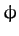--the angles the upper and lower pendulums make with the downward vertical (respectively)--as the generalized coordinates. Do not assume small angles.

3. The surface of the Diskworld is a disk of radius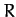which rotates uniformly about a perpendicular axis passing through its center with angular velocity. Diskworld gravitational acceleration is of magnitude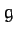, and is everywhere directed normal to the disk. Find the Lagrangian of a projectile of massusing co-rotating cylindrical polar coordinates as the generalized coordinates. What are the momenta conjugate to each coordinate? Are any of these momenta conserved? Find Lagrange's equations of motion for the projectile.

4. Find Lagrange's equations of motion for an elastic pendulum consisting of a particle of massattached to an elastic string of stiffness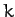and unstretched length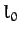. Assume that the motion takes place in a vertical plane.

5. A disk of mass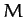and radiusrolls without slipping down a plane inclined at an angleto the horizontal. The disk has a short weightless axle of negligible radius. From this axle is suspended a simple pendulum of length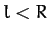whose bob is of mass. Assume that the motion of the pendulum takes place in the plane of the disk. Find Lagrange's equations of motion of the system.

=1.75in

Chapter09/fig9.04.eps
6. A vertical circular hoop of radius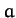is rotated in a vertical plane about a pointon its circumference at the constant angular velocity. A bead of massslides without friction on the hoop. Find the kinetic energy, the potential energy, the Lagrangian, and Largrange's equation of motion of the bead, respectively, in terms of the angular coordinateshown in the above diagram. Here,is a horizontal Cartesian coordinate,a vertical Cartesian coordinate, and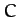the center of the hoop. Show that the beam oscillates like a pendulum about the point on the rim diagrammatically opposite the point about which the hoop rotates. What is the effective length of the pendulum?

7. Consider a spherical pendulum of length. Suppose that the string is initially horizontal, and the bob is rotating horizontally with tangental velocity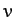. Demonstrate that, at its lowest subsequent point, the bob will have fallen a vertical height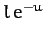, where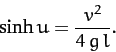Show that ifis large compared to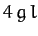then this result becomes approximately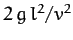.

8. The kinetic energy of a rotating rigid object with an axis of symmetry can be written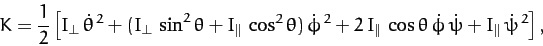where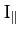is the moment of inertia about the symmetry axis,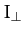is the moment of inertia about an axis perpendicular to the symmetry axis, and,,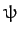are the three Euler angles. Suppose that the object is rotating freely. Find the momenta conjugate to the Euler angles. Which of these momenta are conserved? Find Lagrange's equations of motion for the system. Demonstrate that if the system is precessing steadily (which implies that,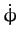, and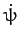are constants) then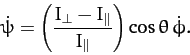9. Consider a nonconservative system in which the dissipative forces take the form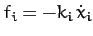, where the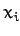are Cartesian coordinates, and the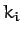are all positive. Demonstrate that the dissipative forces can be incorporated into the Lagrangian formalism provided that Lagrange's equations of motion are modified to read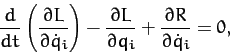where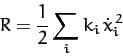is termed the Rayleigh Dissipation Function.Next: Hamiltonian Dynamics Up: Lagrangian Dynamics Previous: Spherical Pendulum
Richard Fitzpatrick 2011-03-31